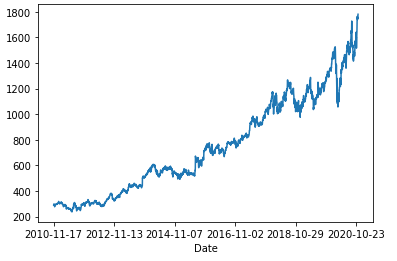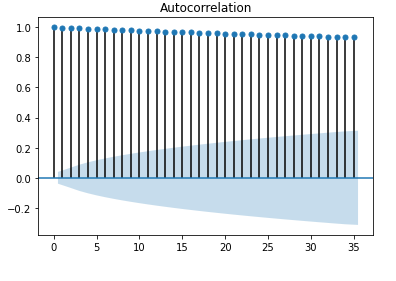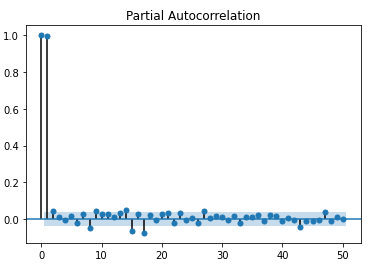# AutoCorrelation

Autocorrelation is the measure of the degree of similarity between a given time series and the lagged version of that time series over successive time periods. It is similar to calculating the correlation between two different variables except in Autocorrelation we calculate the correlation between two different versions Xt and Xt-k of the same time series.

Given time-series measurements, Y1, Y2,…YN at time X1, X2, …XN, the lag k autocorrelation function is defined as:An autocorrelation of +1 represents perfectly positive correlations and -1 represents a perfectly negative correlation.

#### Usage:

• An autocorrelation test is used to detect randomness in the time-series. In many statistical processes, our assumption is that the data generated is random. For checking randomness, we need to check for the autocorrelation of lag 1.
• To determine whether there is a relation between past and future values of time series, we try to lag between different values.

#### Partial Correlation

In time series analysis, the partial autocorrelation function (PACF) gives the partial correlation of a stationary time series with its own lagged values, regressed the values of the time series at all shorter lags. It is different from the autocorrelation function, which does not control other lags.

The formula for calculating PACF at lag k is:where Ti | T(i-1), T(i-2) … T(i-k+1) is the value of residual (error) obtained from fitting a multivariate linear model to T(i-1), T(i-2)…T(i-k+1) for predicting Ti

#### Testing For Autocorrelation

Durbin-Watson Test:

Durbin-Watson test is used to measure the amount of autocorrelation in residuals from the regression analysis. Durbin Watson test is used to check for the first-order autocorrelation.

Assumptions for the Durbin-Watson Test:

• The errors are normally distributed and the mean is 0.
• The errors are stationary.

The test statistics are calculated with the following formula.Where et is the residual of error from the Ordinary Least Squares (OLS) method.

The null hypothesis and alternate hypothesis for the Durbin-Watson Test are

• H0: No first-order autocorrelation.
• H1: There is some first-order correlation.

The Durbin Watson test has values between 0 and 4. Below is the table containing values and their interpretations:

• 2: No autocorrelation. Generally, we assume 1.5 to 2.5 as no correlation.
• 0- <2: positive autocorrelation. The more close it to 0, the more signs of positive autocorrelation.
• >2 -4: negative autocorrelation. The more close it to 4, the more signs of negative autocorrelation.

Python code implementation

 # necessary imports  import pandas as pd  import matplotlib.pyplot as plt  import statsmodels.api as sm  from statsmodels.stats.stattools import durbin_watson  from statsmodels.regression.linear_model import OLS  from statsmodels.graphics.tsaplots import plot_acf , plot_pacf     # Download the google stock last 10 years from Yahoo Finance  goog_stock_Data = pd.read_csv('GOOG.csv', header=0, index_col=0)  goog_stock_Data['Adj Close'].plot()  plt.show()     # Plot the autocorrelation for stock price data with 0.05 significance level  plot_acf(goog_stock_Data['Adj Close'], alpha =0.05)  plt.show()     # Plot the partial autocorrelation for stock price data with   # 0.05 significance level  plot_pacf(goog_stock_Data['Adj Close'], alpha =0.05, lags=50)  plt.show()     """  Code for Durbin Watson test  """ df = pd.DataFrame(goog_stock_Data,columns=['Date','Adj Close'])     X =np.arange(len(df[['Adj Close']]))  Y = np.asarray(df[['Adj Close']])  X = sm.add_constant(X)     # Fit the ordinary least square method.  ols_res = OLS(Y,X).fit()  # apply durbin watson statistic on the ols residual  durbin_watson(ols_res.resid)

Output:

0.027362481492784512• Here, we can see that Durbin-Watson statistics are closer to 0. Hence, there is some positive autocorrelation to the linear model.Autocorrelation plot for different lags

• Above is the autocorrelation plot for different lags. Here, we can see that there is some autocorrelation for significance level 0.05.Partial Autocorrelation graph for different lags

• From the partial autocorrelation, Here, we can see for a 0.05 level of significance there is some partial autocorrelation for the different values of lags. For lag 0 the 100% partial autocorrelation is obvious but for lag 1 also the partial autocorrelation is very high.

#### References:

Whether you're preparing for your first job interview or aiming to upskill in this ever-evolving tech landscape, GeeksforGeeks Courses are your key to success. We provide top-quality content at affordable prices, all geared towards accelerating your growth in a time-bound manner. Join the millions we've already empowered, and we're here to do the same for you. Don't miss out - check it out now!

Previous
Next